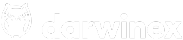CFDs are complex instruments and come with a high risk of losing money rapidly due to leverage. -- % of retail investor accounts lose money when trading CFDs with this provider. You should consider whether you understand how CFDs work and whether you can afford to take the high risk of losing your money.Vol = any volatility indicator reading of your choosing such as ATR
SLmultiplier = an input variable controlling SL size as a multiplier.
TPmultiplier = an input variable controlling TP size as a multiplier.
Risk = an input variable that is the percentage risk of account balance per trade.

I hope the rest are self-explanatory.

``````TPsize = Vol * TPmultiplier
SLsize = Vol * SLmultiplier
SLticks = SLsize / TickSize
AmtToRisk = Risk * 0.01 * AccountBalance
Lotsize = AmtToRisk/SLticks/TickValue``````
7 Likes